Courses

# Test: Runtime Environment

## 20 Questions MCQ Test RRB JE for Computer Science Engineering | Test: Runtime Environment

Description
This mock test of Test: Runtime Environment for Computer Science Engineering (CSE) helps you for every Computer Science Engineering (CSE) entrance exam. This contains 20 Multiple Choice Questions for Computer Science Engineering (CSE) Test: Runtime Environment (mcq) to study with solutions a complete question bank. The solved questions answers in this Test: Runtime Environment quiz give you a good mix of easy questions and tough questions. Computer Science Engineering (CSE) students definitely take this Test: Runtime Environment exercise for a better result in the exam. You can find other Test: Runtime Environment extra questions, long questions & short questions for Computer Science Engineering (CSE) on EduRev as well by searching above.
QUESTION: 1

###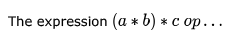where ‘op’ is one of ‘+’, ‘*’ and ‘ ’ (exponentiation) can be evaluated on a CPU with single register without  storing the value of (a * b) if

Solution:

↑ has higer precedence than {*,+,-,/}

So, if op = ↑ implies, we need to evaluate the right hand side of ↑ first and then do the lhs part, which would definately require us to store the value of lhs

but if its a '+' or '*' , we dont need to store the values evaluated, and on the go can do the operation directly on one register.

QUESTION: 2

### The program below uses six temporary variables a,b,c,d,e,f.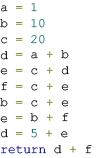Assuming that all operations take their operands from registers, what is the minimum number of registers needed to execute this program without spilling?

Solution:

Here in these types of compiler questions, idea is "map/assign multiple temporaries to one registers."

here a, b, and c all are having 3 different values so i need atleast 3 registers r1, r2 and r3. a is mapped to r1, b to r2 and c to r3

d = a + b, after this line if u notice 'a' is never present on right hand side, so i can map (register of a which is r1 ) d to r1. e = c + d, after this line 'd' is never present on rhs, so i can map (register of d which is r1 ) e to r1.

at this time mapping is

r1 --- e

r2 --- b

r3 --- c

(at this moment i have registers for e, b and c. if i introduce new variable then i may need different register) now at this point if u see

f = c + e

b = c + e

these two are essentially doing same thing, after these two line 'b' and 'f' are same so i can skip computing 'f'. and whereever f is present i will replace it with 'b'. (bcoz neither of 'f' and 'b' are changing after these two lines, so value of these will be 'c+e' forever)

(seems like i introduced one more variable f, and register is needed for that, but actually i did not really introduce 'f'. i am skipping computation of 'f') now at second last line "d = 5 + e" here i introduced 'd', i can map it to any of the register r1 or r3, bcoz after this line neither of 'e' or 'c' is required. (value of 'b' is required bcoz i need to return 'd+f', and 'f' is essentially equal to 'b')

finally code becomes

r1 = 1

r2 = 10

r3 = 20

r1 = r1 + r2

r1 = r3 + r1

(skipping 'f' computation)

r2 = r3 + r1

r2 = r3 + r1

r1 = r2 + r2

r3 = 5 + r1

return r3 + r2

Therefore minimum 3 registers needed.

QUESTION: 3

### Consider evaluating the following expression tree on a machine with load-store architecture in which memory can be accessed only through load and store instructions. The variables  a,b,c,d and e are initially stored in memory. The binary operators used in this expression tree can be evaluated by the machine only when operands are in registers. The instructions produce result only in a register. If no intermediate results can be stored in memory, what is the minimum number of registers needed to evaluate this expression?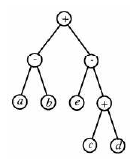Solution:

Given is Load Store Architecture, that means we can access memory using Load and Store Instructions.

Key Idea:- Pick new register only when it is required.

We want to add c and d, and initially both are in memory, therefore copy these into registers.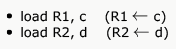(here no compensation can be done, we need two registers)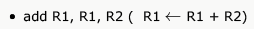(at this point R1 is holding c+d and R2 is holding d, i.e.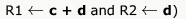Now, e comes into picture and my question is, Can i make use of R1 or R2 to store e ?
I can not use R1 to store e as its value will be needed later but I can use R2.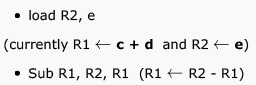Doing this all gives, final value of right sub-tree is stored in R1, and R2 stores e.
Now, coming to left subtree, to perform "a-b" we need to copy both variables in registers.
We can copy one of the variable in R2, but we can not obviously copy in R1 as value of R1 will be required later.

Load R3, b (here comes extra register, and we can not avoid using it.)

Current mapping is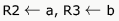and R1 contains final value of Right subtree.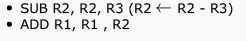Hence 3

*Answer can only contain numeric values
QUESTION: 4

Consider the expression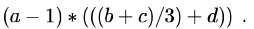Let X be the minimum number of registers required by an optimal code generation (without any register spill) algorithm for a load/store architecture, in which (i) only load and store instructions can have memory operands a n d (ii) arithmetic instructions can have only register or immediate operands. The value of X is _____________ .

Solution: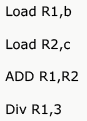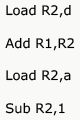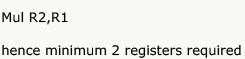QUESTION: 5

In programming languages like C, C++, Python . . . the memory used by a program is typically separated into two parts, the stack and the heap.

Consider the following statements:

1. A stack is efficient for managing nested function calls.

2. Stack space is limited while heap space is not.

3. The stack cannot be used for persistent data structures.

Solution:

Because Size of heap and stack both are limited. There is nothing which is unlimited. Yes but Size of stack and Size of heap is constant. It means that If there is total size available is 10, and If i used 7 block for stack then i can only use 3 block for heap but not more than that. i.e Size of stack and size of heap are inversely proportional. I mean that if size of stack increases then size of heap decreases, and vice versa.

QUESTION: 6

A part of the system software which under all circumstances must reside in the main memory is:

Solution:

The loader is a program that loads the object program from the secondary memory into the main memory for execution of the program. The loader resides in main memory.

QUESTION: 7

A linker is given object modules for a set of programs that were compiled separately. What information need to be included in an object module?

Solution:

(c) is the answer. For linker to link external symbols (for example in C, to link an extern variable in one module to a global variable in another module), it must know the location of all external symbols. In C external symbols includes all global variables and function names.
(a) is trivially there is an object module. (b) must be there if we need to have relocation capability.

QUESTION: 8

A language L   allows declaration of arrays whose sizes are not known during compilation. It is required to make efficient use of memory. Which one of the following is true?

Solution:

If a language L allows declaration of arrays whose sizes are not known during compilation time. It is required to use efficient use of memory.
So a compiler using dynamic memory allocation can be written for L.

An array is a collection of data items, all of the same type, accessed using a common name.

C dynamic memory allocation refers to performing manual memory management for dynamic memory allocation in the C programming language via a group of functions in the C standard library, namely malloc, realloc, calloc and free.

QUESTION: 9

In a resident – OS computer, which of the following systems must reside in the main memory under all situations?

Solution:

In many operating systems the loader is permanently resident in memory, although some operating systems that support virtual memory may allow the loader to be located in a region of memory that is pageable.

QUESTION: 10

Faster access to non-local variables is achieved using an array of pointers to activation records called a

Solution:

properties of displays

1>    Use a pointer array to store the activation records along the static chain.

2>    Fast access for non-local but may be complicated to maintain.

3>    Calling a subprogram in the same level – simply replace and restore.

4>    Calling a subprogram in the higher level – add an entry and may need to save the old pointers.

5>    Calling a subprogram in the lower level – shrink the pointer and restore it when the subprogram returns.

QUESTION: 11

A linker reads four modules whose lengths are 200,800,600,and 500 words, respectively. If they are loaded in that order, what are the relocation constants?

Solution:

first module loaded starting at address 0. Size is 200. hence it will occup first 200 address last address being 199. Second module will be present from 200 and so on.

QUESTION: 12

The process of assigning load addresses to the various parts of the program and adjusting the code and the data in the program to reflect the assigned addresses is called

Solution:

Relocation is the process of assigning load addresses to position-dependent code of a program and adjusting the code and data in the program to reflect the assigned addresses.

Hence Option C is Ans
Symbol resolution  is the process of searching files and libraries to replace symbolic references or names of libraries with actual usable addresses in memory before running a program.

QUESTION: 13

Which one of the following is NOT performed during compilation?

Solution:

Dynamic means- at runtime. Dynamic memory allocation happens during the execution time and hence (A) is the answer.

QUESTION: 14

Consider the following intermediate program in three address code

p = a - b

q = p * c

p = u * v

q = p + q

Which one of the following corresponds to a static single assignment form of the above code?

Solution: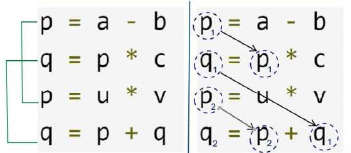QUESTION: 15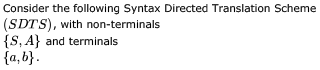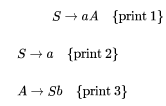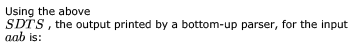Solution:

aab could be derived as follows by the bottom up parser:

S->aA prints 1

A->aSb prints 3

A->aab  prints 2

Now since bottom up parser will work in reverse of right most derivation, so it will print in bottom up fashion i.e., 231 which is option C.

Note that this could also be visualized easily by drawing the derivation tree.

QUESTION: 16

A shift reduce parser carries out the actions specified within braces immediately after reducing with the corresponding rule of grammar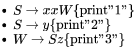What is the translation of xxxxyzz using the syntax directed translation scheme described by the above rules?

Solution:

Making a tree and performing post order traversal will yield answer as A.

S-->x x W (Pf'1')

W-->S z (Pf'3')

S-->x x W (Pf'1')

W-->S z (Pf'3')

S-->y (Pf'2').

QUESTION: 17

In a bottom-up evaluation of a syntax directed definition, inherited attributes can

Solution:

A Syntax Directed Definition (SDD) is called S Attributed if it has only synthesized attributes.

L-Attributed Definitions contain both synthesized and inherited attributes but do not need to build a dependency graph to evaluate them.

QUESTION: 18

Which one of the following is TRUE at any valid state in shift-reduce parsing?

Solution:

A handle is actually the one which is always on the top of the stack. A viable prefix(prefix of the Right-hand side of a production or productions), is actually a prefix of the handle and so can never extend past the right end of the handle(i.e. the top of the stack).
The structure of the stack can be considered as a set of viable prefixes -

QUESTION: 19

Consider the grammar rule E → E1 – E2 for arithmetic expressions. The code generated is targeted to a CPU having a single user register. The subtraction operation requires the first operand to be in the register. If E1 and E2 do not have any common sub expression, in order to get the shortest possible code

Solution:

E2 should be evaluated first

After evaluating E2 first and then E1, we will have E1 in the register and thus we can simply do SUB operation with E2 which will be in memory (as we have only a single register). If we do E1 first and then E2, we must move E2 to memory and E1 back to register before doing SUB, which will increase the code size.

*Answer can only contain numeric values
QUESTION: 20

Consider the following code segment.

x = u - t;

y = x * v;

x = y + w;

y = t - z;

y = x * y;

The minimum number of total variables required to convert the above code segment to static single assignment form is __________.

Solution:

x(temp3) = u(temp1) - t(temp2);

y(temp5) = x * v(temp4);

x(temp7) = y + w(temp6);

y(temp9) = t - z(temp8);

y(temp10) = x * y;